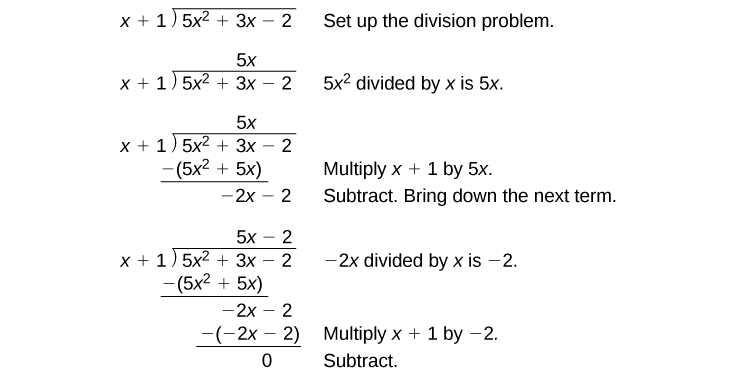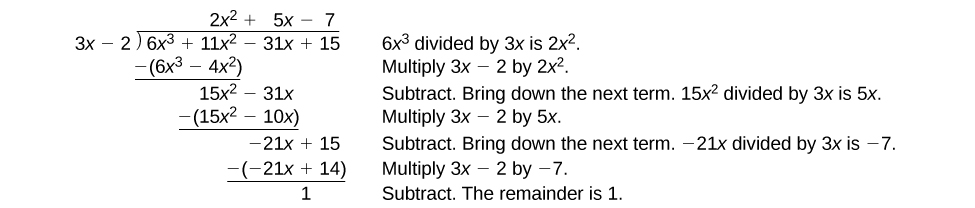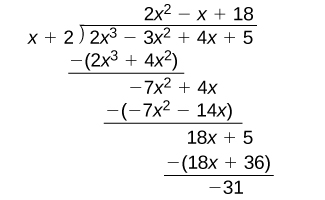5.4 Dividing polynomials  (Page 2/6)

 Page 2 / 6

Given a polynomial and a binomial, use long division to divide the polynomial by the binomial.

1. Set up the division problem.
2. Determine the first term of the quotient by dividing the leading term of the dividend by the leading term of the divisor.
3. Multiply the answer by the divisor and write it below the like terms of the dividend.
4. Subtract the bottom binomial    from the top binomial.
5. Bring down the next term of the dividend.
6. Repeat steps 2–5 until reaching the last term of the dividend.
7. If the remainder is non-zero, express as a fraction using the divisor as the denominator.

Using long division to divide a second-degree polynomial

Divide $\text{\hspace{0.17em}}5{x}^{2}+3x-2\text{\hspace{0.17em}}$ by $\text{\hspace{0.17em}}x+1.$The quotient is $\text{\hspace{0.17em}}5x-2.\text{\hspace{0.17em}}$ The remainder is 0. We write the result as

$\frac{5{x}^{2}+3x-2}{x+1}=5x-2$

or

$5{x}^{2}+3x-2=\left(x+1\right)\left(5x-2\right)$

Using long division to divide a third-degree polynomial

Divide $\text{\hspace{0.17em}}6{x}^{3}+11{x}^{2}-31x+15\text{\hspace{0.17em}}$ by $\text{\hspace{0.17em}}3x-2.\text{\hspace{0.17em}}$There is a remainder of 1. We can express the result as:

$\frac{6{x}^{3}+11{x}^{2}-31x+15}{3x-2}=2{x}^{2}+5x-7+\frac{1}{3x-2}$

Divide $\text{\hspace{0.17em}}16{x}^{3}-12{x}^{2}+20x-3\text{\hspace{0.17em}}$ by $\text{\hspace{0.17em}}4x+5.\text{\hspace{0.17em}}$

$4{x}^{2}-8x+15-\frac{78}{4x+5}$

Using synthetic division to divide polynomials

As we’ve seen, long division of polynomials can involve many steps and be quite cumbersome. Synthetic division is a shorthand method of dividing polynomials for the special case of dividing by a linear factor whose leading coefficient is 1.

To illustrate the process, recall the example at the beginning of the section.

Divide $\text{\hspace{0.17em}}2{x}^{3}-3{x}^{2}+4x+5\text{\hspace{0.17em}}$ by $\text{\hspace{0.17em}}x+2\text{\hspace{0.17em}}$ using the long division algorithm.

The final form of the process looked like this:There is a lot of repetition in the table. If we don’t write the variables but, instead, line up their coefficients in columns under the division sign and also eliminate the partial products, we already have a simpler version of the entire problem.Synthetic division carries this simplification even a few more steps. Collapse the table by moving each of the rows up to fill any vacant spots. Also, instead of dividing by 2, as we would in division of whole numbers, then multiplying and subtracting the middle product, we change the sign of the “divisor” to –2, multiply and add. The process starts by bringing down the leading coefficient.We then multiply it by the “divisor” and add, repeating this process column by column, until there are no entries left. The bottom row represents the coefficients of the quotient; the last entry of the bottom row is the remainder. In this case, the quotient is $\text{\hspace{0.17em}}2{x}^{2}–7x+18\text{\hspace{0.17em}}$ and the remainder is $\text{\hspace{0.17em}}–31.\text{\hspace{0.17em}}$ The process will be made more clear in [link] .

Synthetic division

Synthetic division is a shortcut that can be used when the divisor is a binomial in the form $\text{\hspace{0.17em}}x-k\text{\hspace{0.17em}}$ where $\text{\hspace{0.17em}}k\text{\hspace{0.17em}}$ is a real number. In synthetic division    , only the coefficients are used in the division process.

Given two polynomials, use synthetic division to divide.

1. Write $\text{\hspace{0.17em}}k\text{\hspace{0.17em}}$ for the divisor.
2. Write the coefficients of the dividend.
3. Bring the lead coefficient down.
4. Multiply the lead coefficient by $\text{\hspace{0.17em}}k.\text{\hspace{0.17em}}$ Write the product in the next column.
5. Add the terms of the second column.
6. Multiply the result by $\text{\hspace{0.17em}}k.\text{\hspace{0.17em}}$ Write the product in the next column.
7. Repeat steps 5 and 6 for the remaining columns.
8. Use the bottom numbers to write the quotient. The number in the last column is the remainder and has degree 0, the next number from the right has degree 1, the next number from the right has degree 2, and so on.

what is the period of cos?
your question might not seem clear as you asked. ask well to get perfect answers put your question on a table I'm willing to help you Mr Siyamthemba
Patrick
if tan alpha + beta is equal to sin x + Y then prove that X square + Y square - 2 I got hyperbole 2 Beta + 1 is equal to zero
sin^4+sin^2=1, prove that tan^2-tan^4+1=0
what is the formula used for this question? "Jamal wants to save \$54,000 for a down payment on a home. How much will he need to invest in an account with 8.2% APR, compounding daily, in order to reach his goal in 5 years?"
i don't need help solving it I just need a memory jogger please.
Kuz
A = P(1 + r/n) ^rt
Dale
how to solve an expression when equal to zero
its a very simple
Kavita
gave your expression then i solve
Kavita
Hy guys, I have a problem when it comes on solving equations and expressions, can you help me 😭😭
Thuli
Tomorrow its an revision on factorising and Simplifying...
Thuli
ok sent the quiz
kurash
send
Kavita
Hi
Masum
What is the value of log-1
Masum
the value of log1=0
Kavita
Log(-1)
Masum
What is the value of i^i
Masum
log -1 is 1.36
kurash
No
Masum
no I m right
Kavita
No sister.
Masum
no I m right
Kavita
tan20°×tan30°×tan45°×tan50°×tan60°×tan70°
jaldi batao
Joju
Find the value of x between 0degree and 360 degree which satisfy the equation 3sinx =tanx
what is sine?
what is the standard form of 1
1×10^0
Akugry
Evalute exponential functions
30
Shani
The sides of a triangle are three consecutive natural number numbers and it's largest angle is twice the smallest one. determine the sides of a triangle
Will be with you shortly
Inkoom
3, 4, 5 principle from geo? sounds like a 90 and 2 45's to me that my answer
Neese
answer is 2, 3, 4
Gaurav
prove that [a+b, b+c, c+a]= 2[a b c]
can't prove
Akugry
i can prove [a+b+b+c+c+a]=2[a+b+c]
this is simple
Akugry
hi
Stormzy
x exposant 4 + 4 x exposant 3 + 8 exposant 2 + 4 x + 1 = 0
x exposent4+4x exposent3+8x exposent2+4x+1=0
HERVE
How can I solve for a domain and a codomains in a given function?
ranges
EDWIN
Thank you I mean range sir.
Oliver
proof for set theory
don't you know?
InkoomByBy